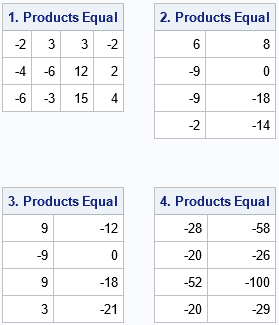I always emphasize efficiency in statistical programming. I have previously written about why you should never multiply with a large diagonal matrix in the SAS IML language. The reason is that it is more efficient to use elementwise multiplication than matrix multiplication. Specifically, if d is a column vector, then I advocate the following tips:

• Instead of diag(d) * A, use d # A to multiply the i_th row of A by the i_th element of d.
• Instead of B * diag(d), use B # d` to multiply the j_th column of B by the j_th element of d.

These tips extend to any matrix. If you need to multiply each column of a matrix by a constant, where the constant is different for each column, use elementwise multiplication:

• If A is a matrix that has n rows and v is a column vector that has n rows, then v#A is the matrix whose i_th column is v[i] times the i_th column of A.
• Similarly, if B is a matrix that has p columns and u is a column vector that has p rows, then u`#B is the matrix whose j_th column is u[j] times the j_th column of B.

Because elementwise multiplication is symmetric, it does not matter whether you write u`#B or B#u`. For convenience, the division operator (/) in IML is overloaded to perform elementwise division, which means that A/v and B/u` are also valid operations. Also, elementwise addition and subtraction are supported, such as A + v and B – u`. For details, see "Shorthand notation for row and column operations."

Elementwise multiplication is also called Hadamard multiplication or the Hadamard product. Whereas books about linear algebra devote many pages to explaining the properties of multiplication by scalars, vectors, and matrices, little (or nothing) is said about the Hadamard product. For reference, this article shows four properties of the Hadamard product that I find useful in computational linear algebra and statistics.

### Four properties of the Hadamard product

In addition to being commutative and associative, the Hadamard product has four properties that are worth listing.

• Let A be an n x p matrix
• Let B be a p x m matrix
• Let v be an n x 1 column vector
• Let u be a p x 1 column vector
• Let w be an m x 1 column vector

The following properties of the Hadamard product (elementwise multiplication) are true. The first property shows how Hadamard multiplication interacts with the matrix transpose operator. The next three properties show how Hadamard multiplication interacts with matrix multiplication:

1. Commutative with transpose: (v#A)` = A`#v` = v`#A`
2. Associative with matrix multiplication on the left: v#(A*B) = (v#A)*B
3. Associative with matrix multiplication on the right: (A*B)#w` = A*(B#w`)
4. Associative with matrix multiplication in the middle: A*(u#B) = (A#u`)*B

I will not show the mathematical proofs for these properties. Rather, I will write a SAS IML program that computes each term in the equation and shows that they are equal. In the following program, n=4, p=3, and m=2.

```/* demonstrate properties of the Hadamard product by running examples */ proc iml; A = {1 2 3, /* 4 x 3 */ -1 2 1, 1 4 5, -1 1 2}; B = {-1 2, /* 3 x 2 */ 2 3, -2 -4}; v = {-2,-3,3,2}; /* 4 x 1 */ u = {-2,-3,3}; /* 3 x 1 */ w = {-3,3}; /* 2 x 1 */   /* 1. (v#A)`= A`#v` = v`#A` */ P1 = (v # A)`; P2 = A` # v`; P3 = v` # A`; if P1=P2 & P2=P3 then print P1[L="1. Products Equal"]; else print "1. Products differ!", P1, P2, P3;   /* 2. v#(A*B) = (v#A)*B */ P1 = v # (A*B); P2 = (v#A)*B; if P1=P2 then print P1[L="2. Products Equal"]; else print "2. Products differ!", P1, P2;   /* 3. (A*B)#w` = A*(B#w`) */ P1 = (A*B) # w`; P2 = A*(B#w`); if P1=P2 then print P1[L="3. Products Equal"]; else print "3. Products differ!", P1, P2;   /* 4. A*(u#B) = (A#u`)*B */ P1 = A*(u#B); P2 = (A#u`)*B; if P1=P2 then print P1[L="4. Products Equal"]; else print "4. Products differ!", P1, P2;```The program shows that all four properties of the Hadamard product are satisfied for the example matrices and vectors.

### Summary

The Hadamard product is a fancy name for elementwise multiplication. You can use the Hadamard product to multiply each column (or row) of a matrix by some constant, where the constant can be different for different rows. To do this, you create a vector that contains the constants. Then a Hadamard product such as v#A multiplies the i_th row of A by the i_th element of v. Similarly, B#u` (or u`#B) multiplies the j_th column of B by the j_th element of u. You can use Hadamard multiplication to avoid matrix multiplications that involve diagonal matrices. Equally useful, you can use Hadamard multiplication to avoid writing a loop that performs a scalar operation over rows or columns.

The SAS IML language supports elementwise operations by using the special elementwise multiplication symbol (#). The IML language supports other elementwise operations, such as division, addition, and subtraction.

The post Properties of the Hadamard product appeared first on The DO Loop.